全國校區一個 Python 報表自動化實戰案例2021-10-25

1.Excel的基本組成
2.一份報表自動化的流程
3.報表自動化實戰
- 當日各項指標同環比情況
- 當日各省份創建訂單量情況
- 最近一段時間創建訂單量趨勢
4.將不同的結果進行合并
- 將不同結果合并到同一個Sheet中
- 將不同結果合并到同一個工作簿的不同Sheet中

Excel的基本組成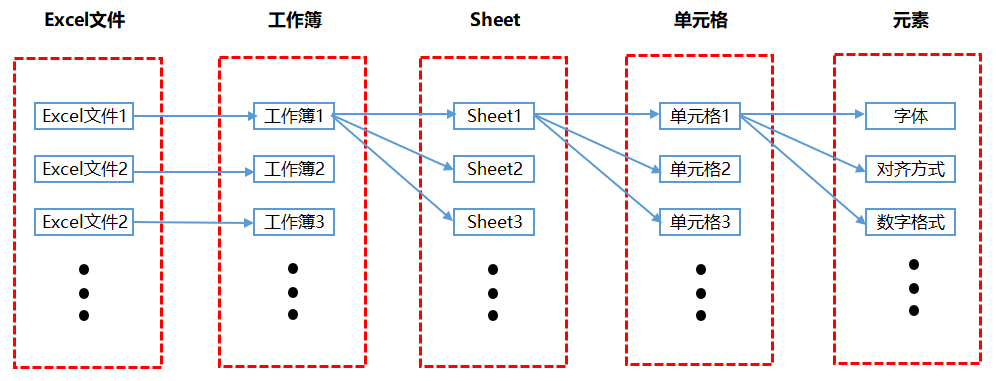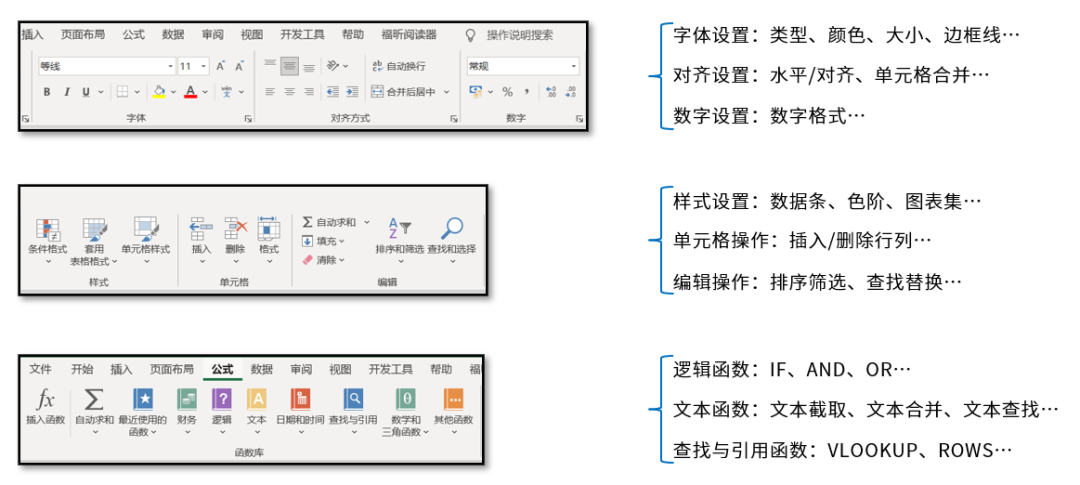一份自動化報表的流程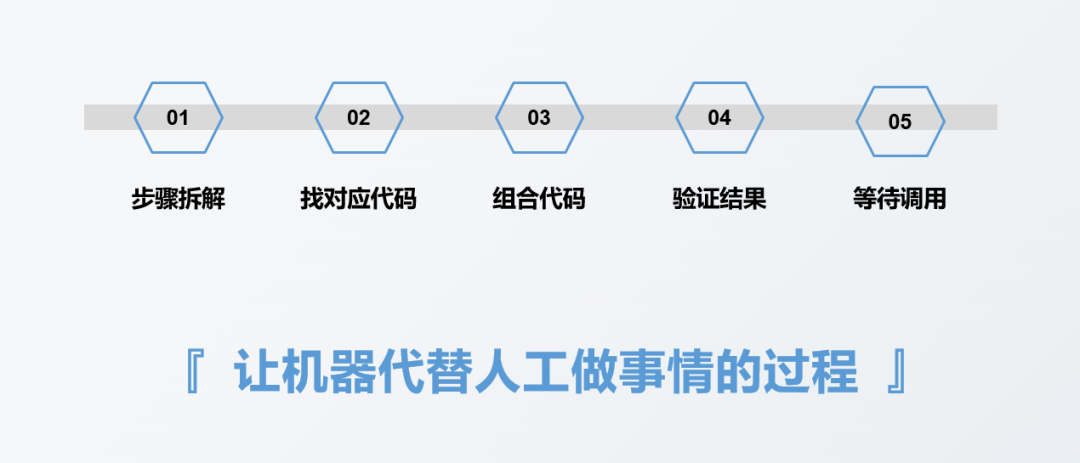報表自動化實戰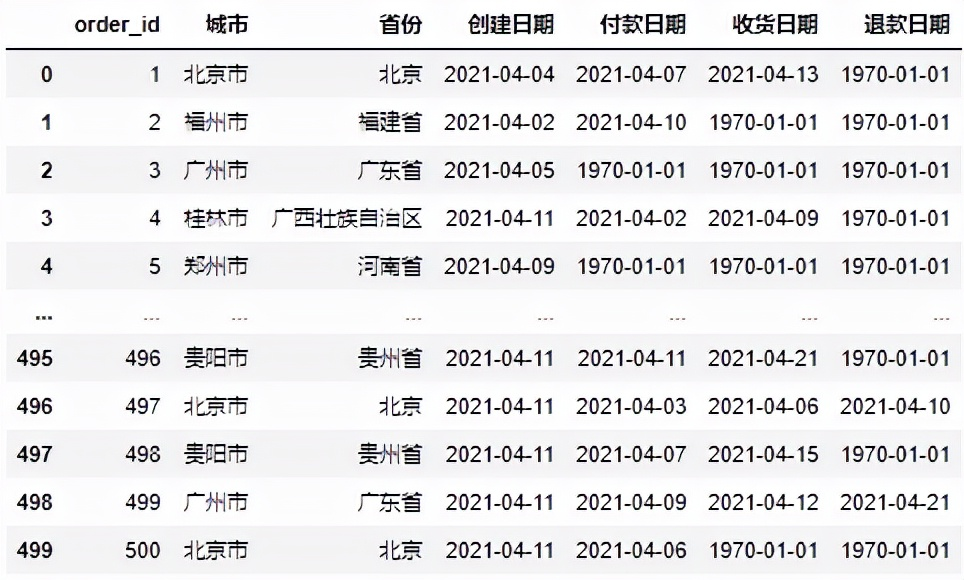• 當日各項指標的同環比情況；
• 當日各省份創建訂單量情況；
• 最近一段時間創建訂單量趨勢

當日各項指標的同環比情況：

#導入文件 import pandas as pd
df = pd.read_excel(r'D:Data-Scienceshareexcel-python報表自動化sale_data.xlsx') #構造同時獲取不同指標的函數 def get_data(date): create_cnt = df[df['創建日期'] == date]['order_id'].count()
pay_cnt = df[df['付款日期'] == date]['order_id'].count()
return_cnt = df[df['退款日期'] == date]['order_id'].count() return create_cnt,pay_cnt,receive_cnt,return_cnt #假設當日是2021-04-11 #獲取不同時間段的各指標值 df_view = pd.DataFrame([get_data('2021-04-11')
,get_data('2021-04-10')
,get_data('2021-04-04')]
,columns = ['創建訂單量','付款訂單量','收貨訂單量','退款訂單量']
,index = ['當日','昨日','上周同期']).T

df_view['環比'] = df_view['當日'] / df_view['昨日'] - 1 df_view['同比'] = df_view['當日'] / df_view['上周同期'] - 1 df_view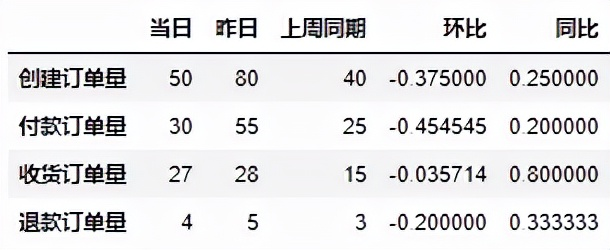from openpyxl import Workbook from openpyxl.utils.dataframe import dataframe_to_rows #創建空工作簿 wb = Workbook()
ws = wb.active #將DataFrame格式數據轉化為openpyxl格式 for r in dataframe_to_rows(df_view,index = True,header = True):
ws.append(r)

wb.save(r'D:Data-Scienceshareexcel-python報表自動化核心指標_原始.xlsx')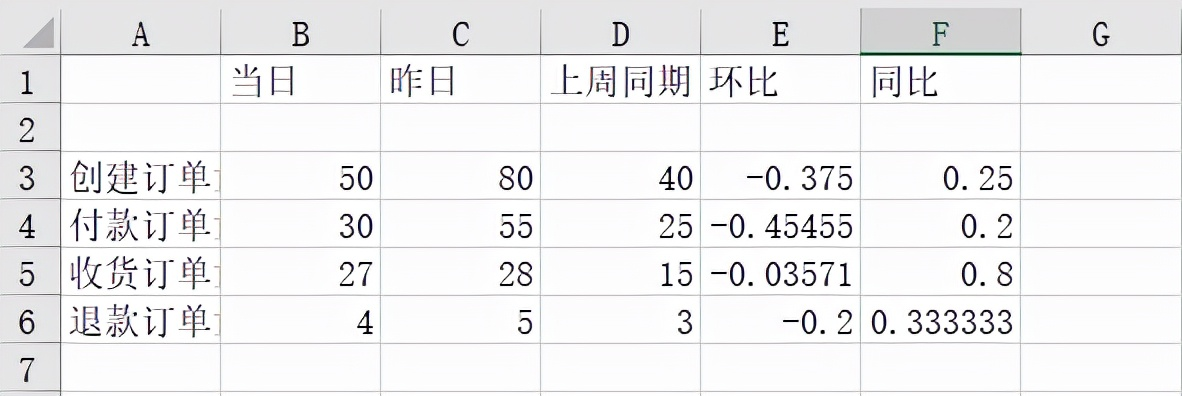from openpyxl import Workbook from openpyxl.utils.dataframe import dataframe_to_rows from openpyxl.styles import colors from openpyxl.styles import Font from openpyxl.styles import PatternFill from openpyxl.styles import Border, Side from openpyxl.styles import Alignment

wb = Workbook()
ws = wb.active for r in dataframe_to_rows(df_view,index = True,header = True):
ws.append(r) #第二行是空的，刪除第二行 ws.delete_rows(2) #給A1單元格進行賦值 ws['A1'] = '指標' #插入一行作為標題行 ws.insert_rows(1)
ws['A1'] = '電商業務方向 2021/4/11 日報' #將標題行的單元格進行合并 ws.merge_cells('A1:F1') #合并單元格 #對第1行至第6行的單元格進行格式設置 for row in ws[1:6]: for c in row: #字體設置 c.font = Font(name = '微軟雅黑',size = 12) #對齊方式設置 c.alignment = Alignment(horizontal = "center") #邊框線設置 c.border = Border(left = Side(border_style = "thin",color = "FF000000"),
right = Side(border_style = "thin",color = "FF000000"),
top = Side(border_style = "thin",color = "FF000000"),
bottom = Side(border_style = "thin",color = "FF000000")) #對標題行和表頭行進行特殊設置 for row in ws[1:2]: for c in row:
c.font = Font(name = '微軟雅黑',size = 12,bold = True,color = "FFFFFFFF")
c.fill = PatternFill(fill_type = 'solid',start_color='FFFF6100') #將環比和同比設置成百分比格式  for col in ws["E":"F"]: for r in col:
r.number_format = '0.00%' #調整列寬 ws.column_dimensions['A'].width = 13 ws.column_dimensions['E'].width = 10 #保存調整后的文件  wb.save(r'D:Data-Scienceshareexcel-python報表自動化核心指標.xlsx')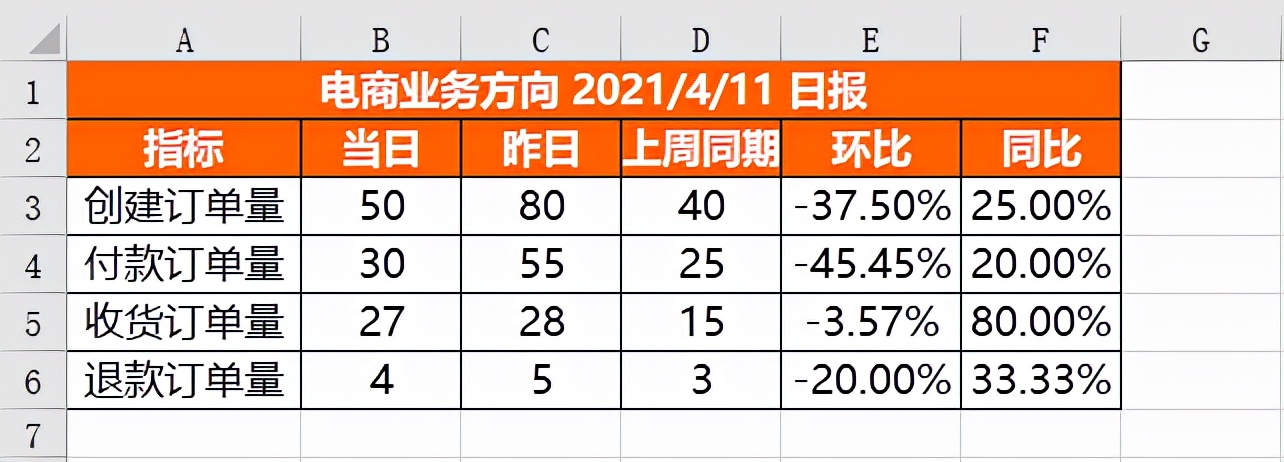當日各省份創建訂單量情況：

df_province = pd.DataFrame(df[df['創建日期'] == '2021-04-11'].groupby('省份')['order_id'].count())
df_province = df_province.reset_index()
df_province = df_province.sort_values(by = 'order_id',ascending = False)
df_province = df_province.rename(columns = {'order_id':'創建訂單量'})
df_province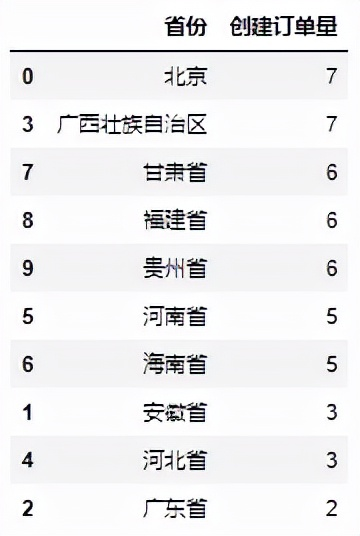from openpyxl import Workbook from openpyxl.utils.dataframe import dataframe_to_rows from openpyxl.styles import colors from openpyxl.styles import Font from openpyxl.styles import PatternFill from openpyxl.styles import Border, Side from openpyxl.styles import Alignment from openpyxl.formatting.rule import DataBarRule

wb = Workbook()
ws = wb.active for r in dataframe_to_rows(df_province,index = False,header = True):
ws.append(r) #對第1行至第11行的單元格進行設置 for row in ws[1:11]: for c in row: #字體設置 c.font = Font(name = '微軟雅黑',size = 12) #對齊方式設置 c.alignment = Alignment(horizontal = "center") #邊框線設置 c.border = Border(left = Side(border_style = "thin",color = "FF000000"),
right = Side(border_style = "thin",color = "FF000000"),
top = Side(border_style = "thin",color = "FF000000"),
bottom = Side(border_style = "thin",color = "FF000000")) #設置進度條條件格式 rule = DataBarRule(start_type = 'min',end_type = 'max',
color="FF638EC6", showValue=True, minLength=None, maxLength=None)
ws.conditional_formatting.add('B1:B11',rule) #對第1行標題行進行設置 for c in ws:
c.font = Font(name = '微軟雅黑',size = 12,bold = True,color = "FFFFFFFF")
c.fill = PatternFill(fill_type = 'solid',start_color='FFFF6100') #調整列寬 ws.column_dimensions['A'].width = 17 ws.column_dimensions['B'].width = 13 #保存調整后的文件  wb.save(r'D:Data-Scienceshareexcel-python報表自動化各省份銷量情況.xlsx')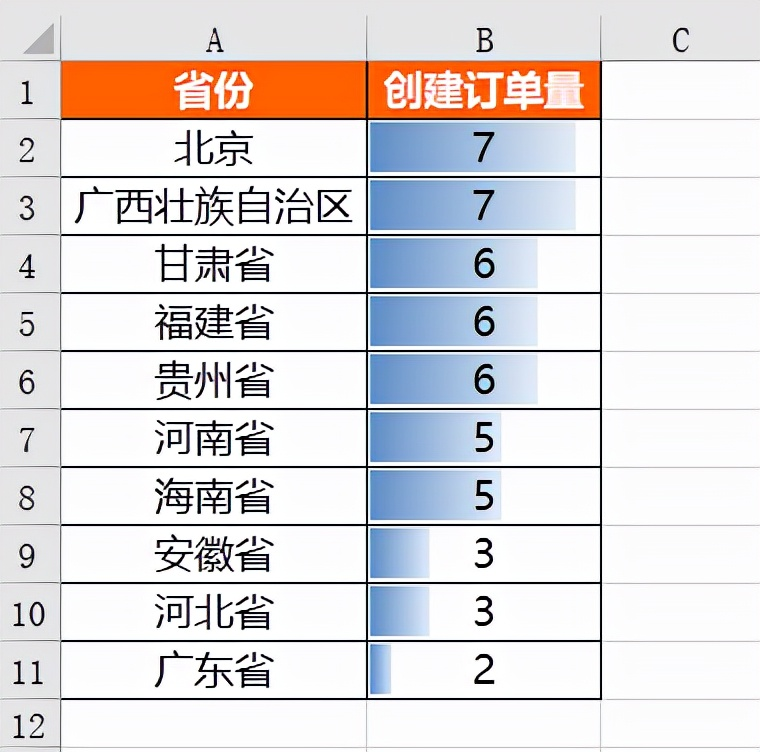最近一段時間創建訂單量趨勢：

%matplotlib inline import matplotlib.pyplot as plt
plt.rcParams["font.sans-serif"]='SimHei'#解決中文亂碼 #設置圖表大小 plt.figure(figsize = (10,6))
df.groupby('創建日期')['order_id'].count().plot()
plt.title('4.2 - 4.11 創建訂單量分日趨勢')
plt.xlabel('日期')
plt.ylabel('訂單量') #將圖表保存到本地 plt.savefig(r'D:Data-Scienceshareexcel-python報表自動化4.2 - 4.11 創建訂單量分日趨勢.png')

from openpyxl import Workbook from openpyxl.drawing.image import Image

wb = Workbook()
ws = wb.active

img = Image(r'D:Data-Scienceshareexcel-python報表自動化4.2 - 4.11 創建訂單量分日趨勢.png')

wb.save(r'D:Data-Scienceshareexcel-python報表自動化4.2 - 4.11 創建訂單量分日趨勢.xlsx')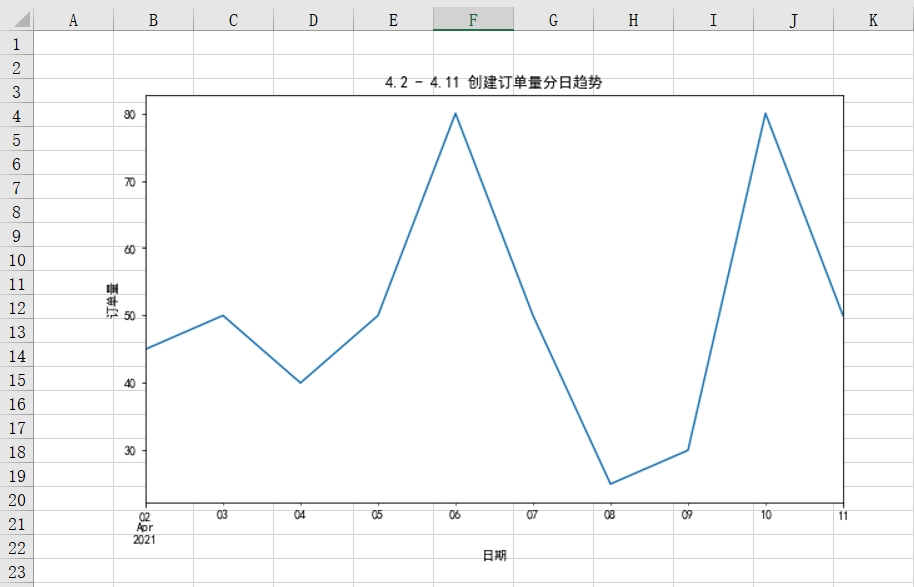將不同的結果合并到同一個Sheet中：

for r in dataframe_to_rows(df_view,index = True,header = True): ws.append(r)

for j in range(df_province.shape):
ws.cell(row = df_view.shape + 5,column = 1 + j).value = df_province.columns[r]

df_province.shape是獲取df_province表有多少列，df_view.shape是獲取df_view表有多少行。

#再把具體的值插入 for i in range(df_province.shape): for j in range(df_province.shape):
ws.cell(row = df_view.shape + 6 + i,column = 1 + j).value = df_province.iloc[i,j]

#插入圖片 img = Image(r'D:Data-Scienceshareexcel-python報表自動化4.2 - 4.11 創建訂單量分日趨勢.png')

#格式預設 #表頭字體設置 title_Font_style = Font(name = '微軟雅黑',size = 12,bold = True,color = "FFFFFFFF") #普通內容字體設置 plain_Font_style = Font(name = '微軟雅黑',size = 12)
Alignment_style = Alignment(horizontal = "center")
Border_style = Border(left = Side(border_style = "thin",color = "FF000000"),
right = Side(border_style = "thin",color = "FF000000"),
top = Side(border_style = "thin",color = "FF000000"),
bottom = Side(border_style = "thin",color = "FF000000"))
PatternFill_style = PatternFill(fill_type = 'solid',start_color='FFFF6100')

#對A1至F6范圍內的單元格進行設置 for row in ws['A1':'F6']: for c in row:
c.font = plain_Font_style
c.alignment = Alignment_style
c.border = Border_style #對第1行和第2行的單元格進行設置 for row in ws[1:2]: for c in row:
c.font = title_Font_style
c.fill = PatternFill_style #對E列和F列的單元格進行設置 for col in ws["E":"F"]: for r in col:
r.number_format = '0.00%' #對A9至B19范圍內的單元格進行設置 for row in ws['A9':'B19']: for c in row:
c.font = plain_Font_style
c.alignment = Alignment_style
c.border = Border_style #對A9至B9范圍內的單元格進行設置 for row in ws['A9':'B9']: for c in row:
c.font = title_Font_style
c.fill = PatternFill_style #設置進度條 rule = DataBarRule(start_type = 'min',end_type = 'max',
color="FF638EC6", showValue=True, minLength=None, maxLength=None)
ws.conditional_formatting.add('B10:B19',rule) #調整列寬 ws.column_dimensions['A'].width = 17 ws.column_dimensions['B'].width = 13 ws.column_dimensions['E'].width = 10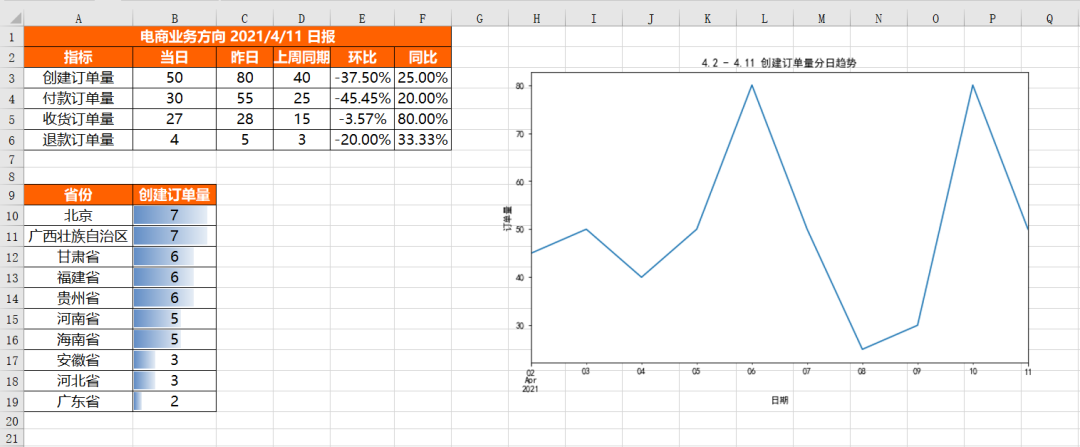將不同的結果合并到同一工作簿的不同Sheet中：

from openpyxl import Workbook from openpyxl.utils.dataframe import dataframe_to_rows

wb = Workbook()
ws = wb.active

ws1 = wb.create_sheet()
ws2 = wb.create_sheet() #更改sheet的名稱 ws.title = "核心指標" ws1.title = "各省份銷情況" ws2.title = "分日趨勢" for r1 in dataframe_to_rows(df_view,index = True,header = True):
ws.append(r1) for r2 in dataframe_to_rows(df_province,index = False,header = True):
ws1.append(r2)

img = Image(r'D:Data-Scienceshareexcel-python報表自動化4.2 - 4.11 創建訂單量分日趨勢.png')

wb.save(r'D:Data-Scienceshareexcel-python報表自動化多結果合并_多Sheet.xlsx')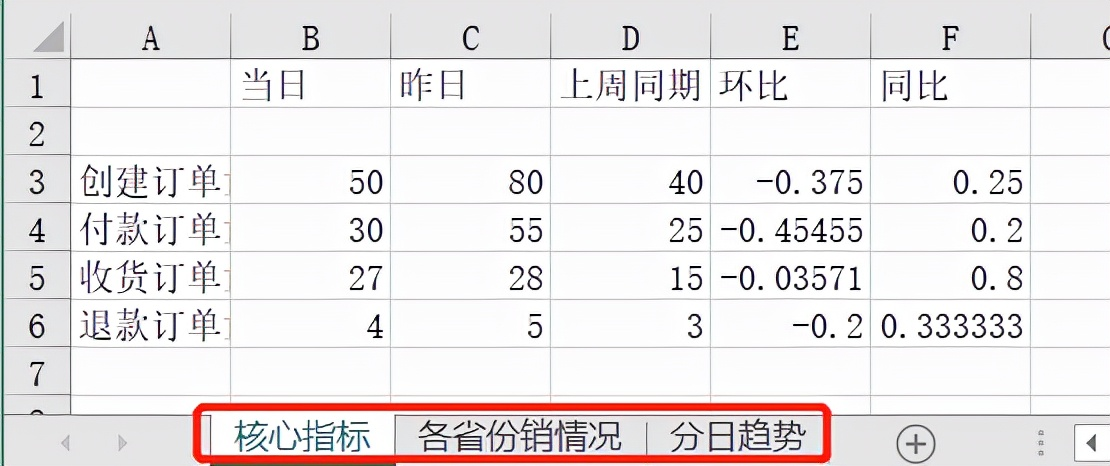OK